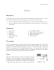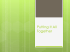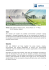# Ms. Mulrooney Physics Worksheet: Coefficients of

## Transcription

Ms. Mulrooney Physics Worksheet: Coefficients of
```Ms. Mulrooney
Physics
Worksheet: Coefficients of Friction and Overcoming Friction
1. A bag of sugar has a mass of 2.26 kg.
a. What is its weight in newtons on the moon, where the acceleration due to gravity is
one-sixth that on Earth?
b. What is its weight on Jupiter, where the acceleration due to gravity is 2.64 times that
on Earth?
2. A 2.0 kg block on an incline at 60.0˚ angle is held in equilibrium by a horizontal force.
a. Determine the magnitude of this horizontal force (disregard friction).
b. Determine the magnitude of the normal force on the block.
3. A 24 kg crate at rest on a horizontal floor requires a 75 N horizontal force to set it in motion.
Once it is in motion, a horizontal force of 53 N keeps it moving with a constant velocity.
a. Find the coefficient of static friction between the crate and the floor.
b. Find the coefficient of kinetic friction between the crate and the floor.
4. A 25 kg chair initially at rest on a horizontal floor requires a 165 N horizontal force to set it
in motion. Once the chair is in motion, a 127 N horizontal force keeps it moving at constant
velocity.
a. Find the coefficient of static friction between the chair and the floor.
b. Find the coefficient of kinetic friction between the chair and the floor.
5. A student pulls on a rope attached to a box of books and moves the box down the hall. The
student pulls with a force of 185 N at an angle of 25.0˚ above the horizontal. The box has a
mass of 35.0 kg and μk between the box and the floor is 0.27. Find the acceleration of the
box.
6. A 75 kg kg box slides down a 25.0˚ ramp with an acceleration of 3.602 m/s2.
a. Find μk between the box and the ramp.
b. What acceleration would a 175 kg box have on this ramp?
7. A box of books weighing 325N moves at a constant velocity across the floor when the box is
pushed with a force of 425N exerted downward at an angle of 35.2˚ below the horizontal.
Find μk between the box and the floor.
8. The force of air resistance acting on a certain falling object is roughly proportional to the
square of the object’s velocity and is directed upward. If the object falls fast enough, will the
force of air resistance eventually exceed the weight of the object and cause the object to
move upward? Explain.
```

### Free-Body Diagram Worksheet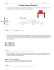### Practice problems for recitation â Exam II 1. A 50.0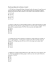### P. LeClair - The University of Alabama### Past Final Exam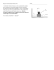### Vector Components Worksheet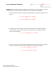### Chapter 5 Review Physics 11 1.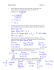### Practice(Exam(II( 1.#What#is#the#maximum#speed#a#car#can#go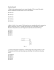### Exam 2 Solutions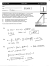### Sample Final Exam Physics 131 Spring 2009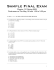### Solving Literal Equations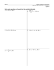### Projectile Motion Worksheet (Case 1)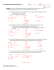### SAMPLE FINAL Name:____________________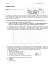### Exam 1 Practice Problems 1. (hr02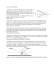### Physics Worksheet Lesson 10 Newton's Third Law of Motion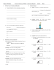### GO ON TO THE NEXT PAGE. Section I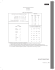### Phys 111 CE1 2013 Fall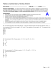### Sample Final Exam #2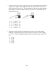### A Worksheet not slide backwards relative to the train? 8) A 1.20 x 10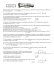### Practice Exam #6 - Mr. Britton / FHS Physics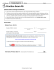### Friction - Mississippi State Physics Labs